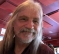## Aliasing in Radix-2 FFT - Decimation in timeStarted by 4 years ago7 replieslatest reply 4 years ago156 views

Hi,

We know for Radix-2 FFT, an N-point DFT is broken down to multiple 2-point DFTs. In doing so, one of the methods is to decimate in time, i.e., subsequent downsampling of the input by a factor of 2.

From the sampling theory, we know that for a donwsmapling by a factor of M, we need signal bandwidth omega <= pi/M to avoid aliasing.

So, the question is, why the subsequent downsampling in the FFT algorithm doesn't cause aliasing even though we don't use any pre-filtering? I believe it's due to the fact that with each downsampling, we also reduce the DFT point to half (i.e., when we downsample by a factor of 2, we also reduce the DFT point from N to N/2), but I am having trouble in explaining this using math.

[ - ]Similarly to some filterbank and wavelet transforms, there is perfect reconstruction because the aliases have a way of killing each other off.

But when thinking about the DFT, don't think of it as the Fourier Transform of a bandlimited signal in continuous time.  Instead think of it as exactly the same as the Discrete Fourier Series.  The DFT maps a discrete and periodic (with period N) sequence of numbers from one domain (let's say the "time domain") to another discrete and periodic sequence in the reciprocal domain ("frequency domain") having the same period.  There is no issue of aliasing with the DFT, but there might be an issue of aliasing regarding some operations of the DFT, such as circular convolution in one domain (which corresponds to multiplication in the reciprocal domain).

take a look at this Stack Exchange:

and this

[ - ]Thanks rbj. I totally agree to your SE post. I also would like to think DFT exactly in the same way as DFS because the modular arithmetic related to DFT essentially makes the sequence exactly the same periodic sequence of DFS in terms of handling.

But, we also know that DFT (or DFS) can be calculated by sampling the DTFT at omega = 2 pi k / N. This is where it is a bit confusing to me. If the DTFT of a sequence has a bandwidth omega_max > pi/2, a downsampling by a factor of 2 is surely going to cause aliasing in the resulting DTFT. Therefore, the DFTs we get by sampling the original DTFT and downsampled DTFT should differ (due to aliasing).

But the radix FFT is telling us that, if we do the same downsampling and perform DFT directly, there will be no aliasing error. What am I missing here?

[ - ]I think it may be a semantic issue with some poor nomenclature in this case.

"Decimation" in the context of FFTs isn't downsampling.  i.e., "decimation" in time or frequency for an FFT just tells you whether the algorithm does the bit-reversal process first or last.   It's not really downsampling the signal anywhere, at least it is not necessary to think of it that way.

Remember the FFT is just an algorithm that exploits a factorization of the DFT matrix.   The DFT does no downsampling, and can be thought of as an N-stage correlator against a set of N reference functions that are orthogonal frequencies of sinusoids.  The factorization doesn't really force any downsampling, it just utilizes a handy order of computing all the outputs.

I hope that helps a little bit.   I think you're not seeing the downsampling because it isn't actually there.  ;)

[ - ]Thanks a lot, . I get what you are saying but by confusion was, well, at the end we are calculating DFTs of a new sequence constructed using the alternate samples of the original sequence. That's the textbook definition of downsampling by 2, right? E.g., if we take only the even branch of the FFT, we will surely get the aliasing effect, I believe.

So, I think you and rbj are meaning the same thing, when we take even and odd branch, perform DFT, and then combine, the resulting effect nullifies the aliasing error. In that sense, decimation in FFT is not same as downsampling the signal.

Thanks again for your inputs. It helped me do some thinking on this topic.

[ - ]"If the DTFT of a sequence has a bandwidth omega_max > pi/2, a downsampling by a factor of 2 is surely going to cause aliasing in the resulting DTFT."

Yes it will.  So kicking out all of the odd-indexed samples will cause aliasing.  So also will kicking out all of the even-indexed samples.  That will also cause aliasing.

But aliasing is deterministic.  It is not random noise.  The math turns out that the aliasing you get from removing the odd-indexed samples will be cancelled by the aliasing you get from removing the even-indexed samples.

Consider a general sequence x[n].  Removing the odd-indexed samples results in

x_e[n] = x[n](1 + (-1)^n)/2

and removing the even-indexed samples results in

x_o[n] = x[n](1 - (-1)^n)/2

So now what happens when you add x_e[n] to x_o[n]?

[ - ]Thanks a lot rbj, that actually makes sense.

[ - ]